# 迹忆客专注技术分享

## 排序算法学习之路——选择排序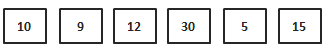v = 10 ，p = 0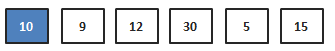v = 12 ，p = 2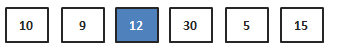v = 30 ，p = 3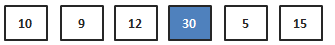v = 10 ， p = 0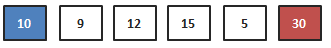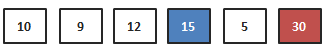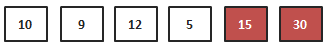15和30一样也不再参与排序。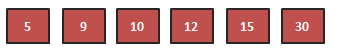1）首先在未排序序列中找到最小（大）元素，存放到排序序列的起始位置
2）再从剩余未排序元素中继续寻找最小（大）元素，然后放到已排序序列的末尾。
3）重复第二步，直到所有元素均排序完毕。

``````/**
* 交换函数
*/
function swap(&\$arr,\$a,\$b){
\$t = \$arr[\$a];
\$arr[\$a] = \$arr[\$b];
\$arr[\$b] = \$t;
}
function SelectSort(&\$arr){
\$end = count(\$arr)-1;
do{
\$p = 0;
for(\$i=0;\$i<=\$end;\$i++){
if(\$arr[\$i]>\$arr[\$p]){
\$p = \$i;
}
}
swap(\$arr,\$p,\$end);
}while(--\$end>0);
}
\$arr = array(10,6,8,23,4,1,17,56,32,50,11,9);
SelectSort(\$arr);
print_r(\$arr);
``````## 热门标签